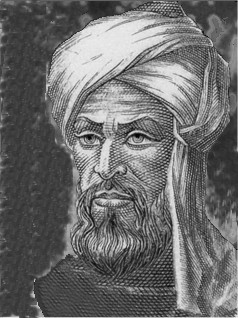Algebra Seminar

• M 4:00-5:00pm
• UH 4170

Next Talk

• February 18, 2019
• Alessandro Arsie
• Sheaves and Algebraic Geometry
• Abstract: I will review the definitions of presheaf and sheaf, give some examples, and talk about the sheafification, morphisms of sheaves, short exact sequences, Cech cohomology with value in a sheaf of Abelian groups (say) and the induced long exact sequence. Then if I have time (but probably I will have to postpone it to another occasion) I can show that the Picard group of complex manifold $X$ (group of holomorphic line bundles modulo isomorphism, with groups structure given by tensor product) is isomorphic to $H^1(X, O^*)$ the first cohomology group with values in the sheaf $O^*$ of nowhere zero holomorphic functions.

Talks This Semester

• February 18, 2019
• Alessandro Arsie
• Sheaves and Algebraic Geometry
• Abstract: I will review the definitions of presheaf and sheaf, give some examples, and talk about the sheafification, morphisms of sheaves, short exact sequences, Cech cohomology with value in a sheaf of Abelian groups (say) and the induced long exact sequence. Then if I have time (but probably I will have to postpone it to another occasion) I can show that the Picard group of complex manifold $X$ (group of holomorphic line bundles modulo isomorphism, with groups structure given by tensor product) is isomorphic to $H^1(X, O^*)$ the first cohomology group with values in the sheaf $O^*$ of nowhere zero holomorphic functions.

• January 28, 2019
• Alessandro Arsie
• Sheaves and Algebraic Geometry
• Abstract: I will review the definitions of presheaf and sheaf, give some examples, and talk about the sheafification, morphisms of sheaves, short exact sequences, Cech cohomology with value in a sheaf of Abelian groups (say) and the induced long exact sequence. Then if I have time (but probably I will have to postpone it to another occasion) I can show that the Picard group of complex manifold $X$ (group of holomorphic line bundles modulo isomorphism, with groups structure given by tensor product) is isomorphic to $H^1(X, O^*)$ the first cohomology group with values in the sheaf $O^*$ of nowhere zero holomorphic functions.